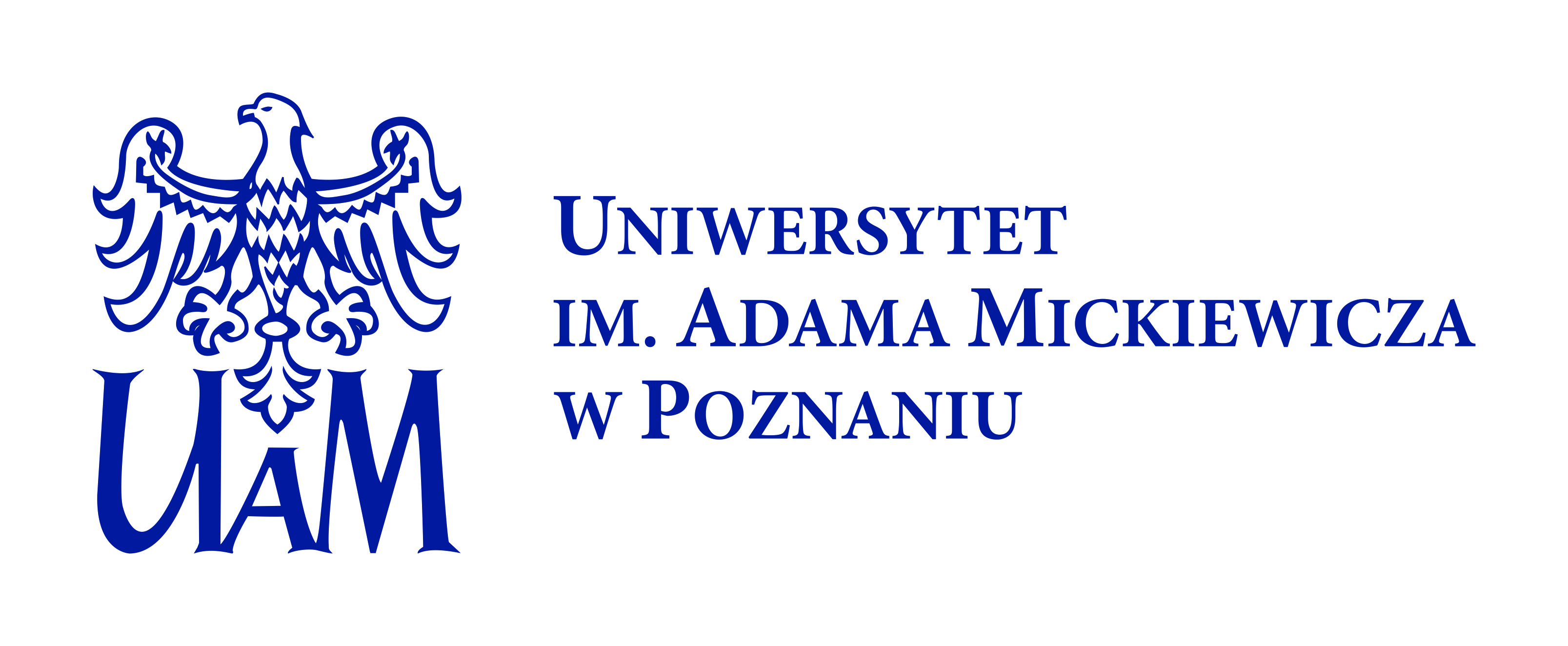# Comparison of values of Pearson’s and Spearman’s correlation coefficient on the same sets of data2011

## Publisher

Wydział Nauk Geograficznych i Geologicznych Uniwersytetu im. Adama Mickiewicza

## Abstract

Spearman’s rank correlation coefficient is a nonparametric (distribution-free) rank statistic proposed by Charles Spearman as a measure of the strength of an association between two variables. It is a measure of a monotone association that is used when the distribution of data makes Pearson’s correlation coefficient undesirable or misleading. Spearman’s coefficient is not a measure of the linear relationship between two variables, as some “statisticians” declare. It assesses how well an arbitrary monotonic function can describe a relationship between two variables, without making any assumptions about the frequency distribution of the variables. Unlike Pearson’s product-moment correlation coefficient, it does not require the assumption that the relationship between the variables is linear, nor does it require the variables to be measured on interval scales; it can be used for variables measured at the ordinal level. The idea of the paper is to compare the values of Pearson’s product-moment correlation coefficient and Spearman’s rank correlation coefficient as well as their statistical significance for different sets of data (original - for Pearson’s coefficient, and ranked data for Spearman’s coefficient) describing regional indices of socio-economic development.

## Keywords

Pearson’s correlation coefficient, Spearman’s rank correlation coefficient, Kendall’s tau, regional indices of socio-economic development

## Citation

Quaestiones Geographicae vol. 30 (2), 2011, pp. 87-93

## Collections